## CosineLetbe an Angle measured counterclockwise from the-axis along the arc of the unit Circle. Thenis the horizontal coordinate of the arc endpoint. As a result of this definition, the cosine function is periodic with period.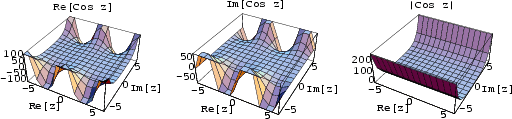The cosine function has a Fixed Point at 0.739085.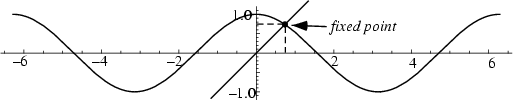The cosine function can be defined algebraically using the infinite sum(1)

or the Infinite Product(2)

A close approximation tofor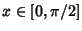is(3)

(Hardy 1959). The difference betweenand Hardy's approximation is plotted below.The Fourier Transform ofis given by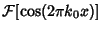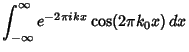(4)

whereis the Delta Function.

The cosine sum rule gives an expansion of the Cosine function of a multiple Angle in terms of a sum of Powers of sines and cosines,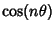(5)

Summing the Cosine of a multiple angle from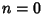tocan be done in closed form using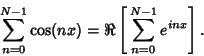(6)

The Exponential Sum Formulas give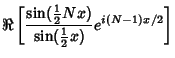(7)

Similarly,(8)

where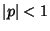. The Exponential Sum Formula gives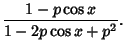(9)

Cvijovic and Klinowski (1995) note that the following series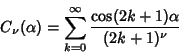(10)

has closed form for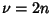,(11)

whereis an Euler Polynomial.

See also Euler Polynomial, Exponential Sum Formulas, Fourier Transform--Cosine, Hyperbolic Cosine, Sine, Tangent, Trigonometric Functions

References

Abramowitz, M. and Stegun, C. A. (Eds.). Circular Functions.'' §4.3 in Handbook of Mathematical Functions with Formulas, Graphs, and Mathematical Tables, 9th printing. New York: Dover, pp. 71-79, 1972.

Hardy, G. H. Ramanujan: Twelve Lectures on Subjects Suggested by His Life and Work, 3rd ed. New York: Chelsea, p. 68, 1959.

Cvijovic, D. and Klinowski, J. Closed-Form Summation of Some Trigonometric Series.'' Math. Comput. 64, 205-210, 1995.

Hansen, E. R. A Table of Series and Products. Englewood Cliffs, NJ: Prentice-Hall, 1975.

Project Mathematics! Sines and Cosines, Parts I-III. Videotapes (28, 30, and 30 minutes). California Institute of Technology. Available from the Math. Assoc. Amer.

Spanier, J. and Oldham, K. B. The Sineand CosineFunctions.'' Ch. 32 in An Atlas of Functions. Washington, DC: Hemisphere, pp. 295-310, 1987.